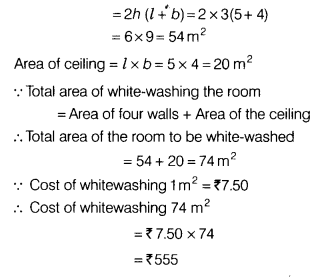# The length, breadth and height of a room are 5 m, 4 m and 3 m, respectively. Find the cost of white-washing the walls of the room and the ceiling at the rate of f. 7.50 per sq m

The length, breadth and height of a room are 5 m, 4 m and 3 m, respectively. Find the cost of white-washing the walls of the room and the ceiling at the rate of f. 7.50 per sq m.

Given, length of a room, l = 5 m
breadth of a room, fa = 4m
and height of a room, h = 3 m
∴ Area of four walls of the room